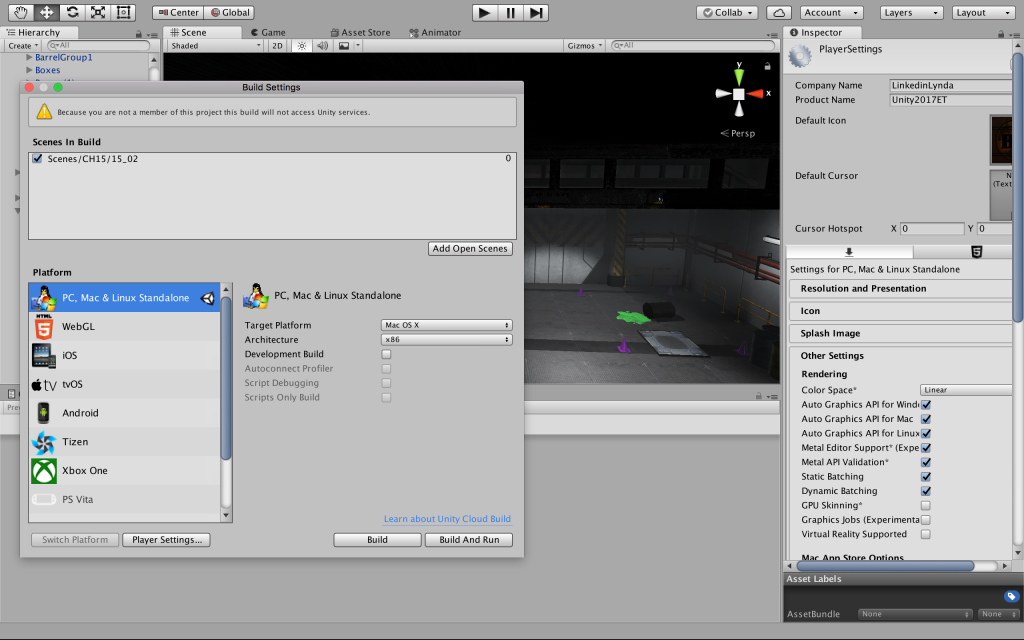# joxin-UnityEssentials

Notes for myself:

1. Before turning an object into a prefab, I should place it in a desired position in relation to the origin (aka the transform information of the prefabs should have the desired position when it is placed at [0, 0, 0]).
2. light probe group not working on my machine?
3. Use q, w, e, r, t to toggle between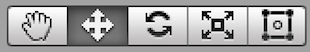.

Tutorial_1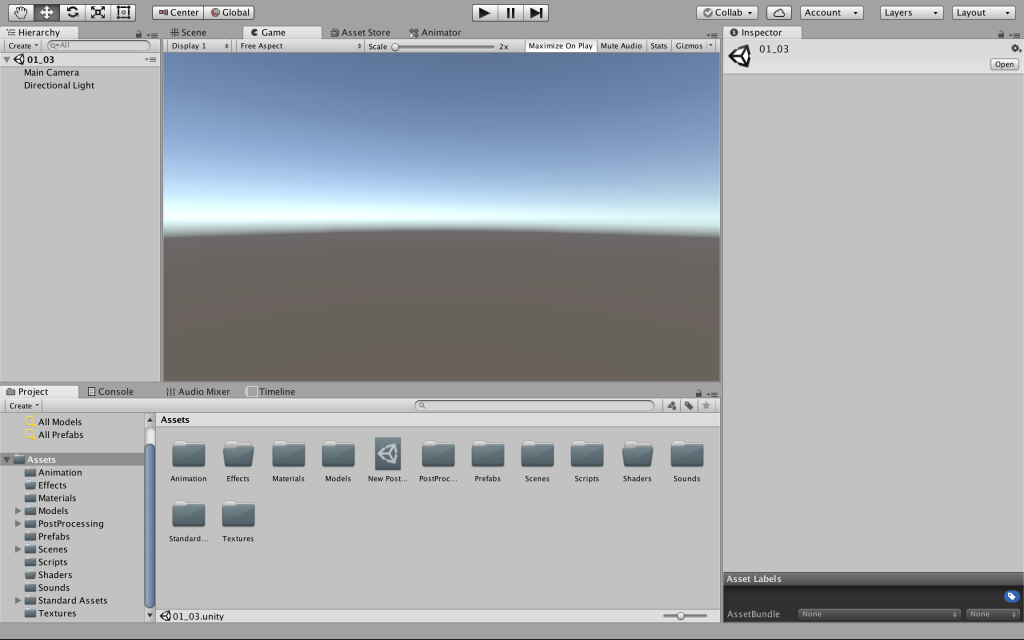Tutorial_2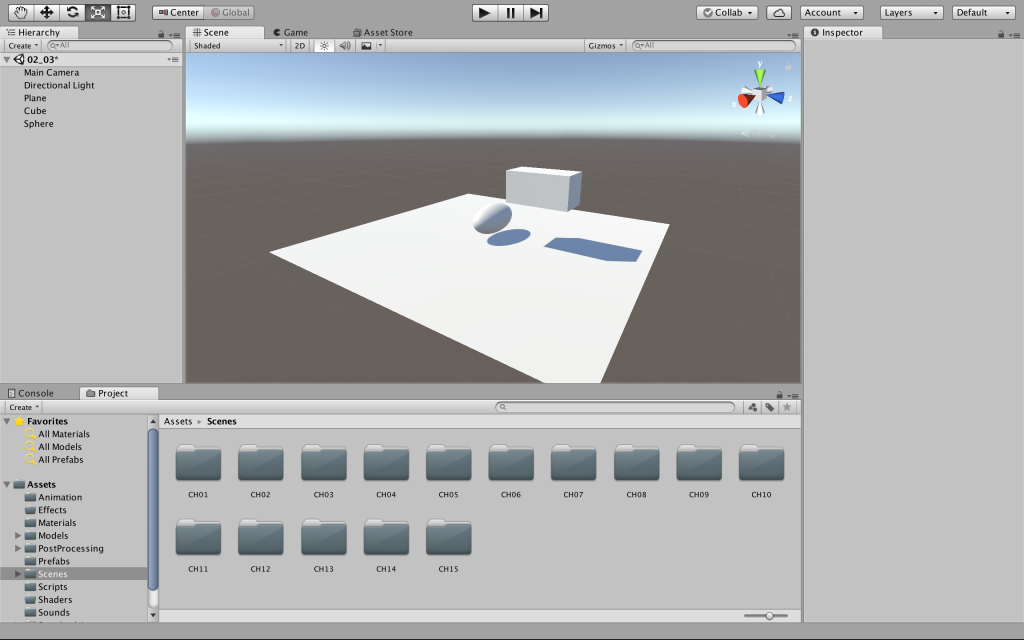Tutorial_3Tutorial_4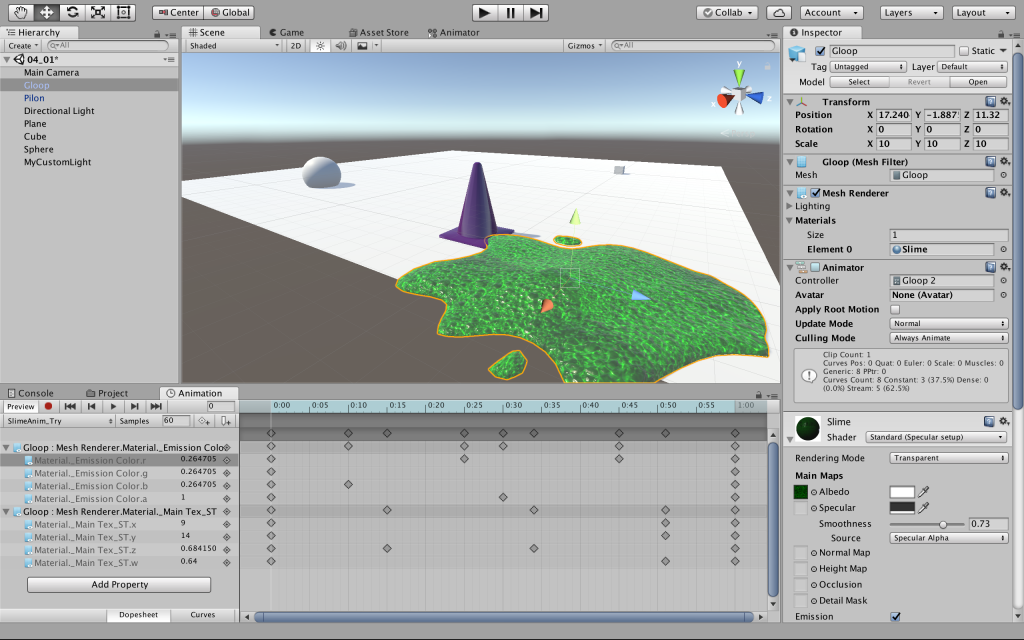Tutorial_5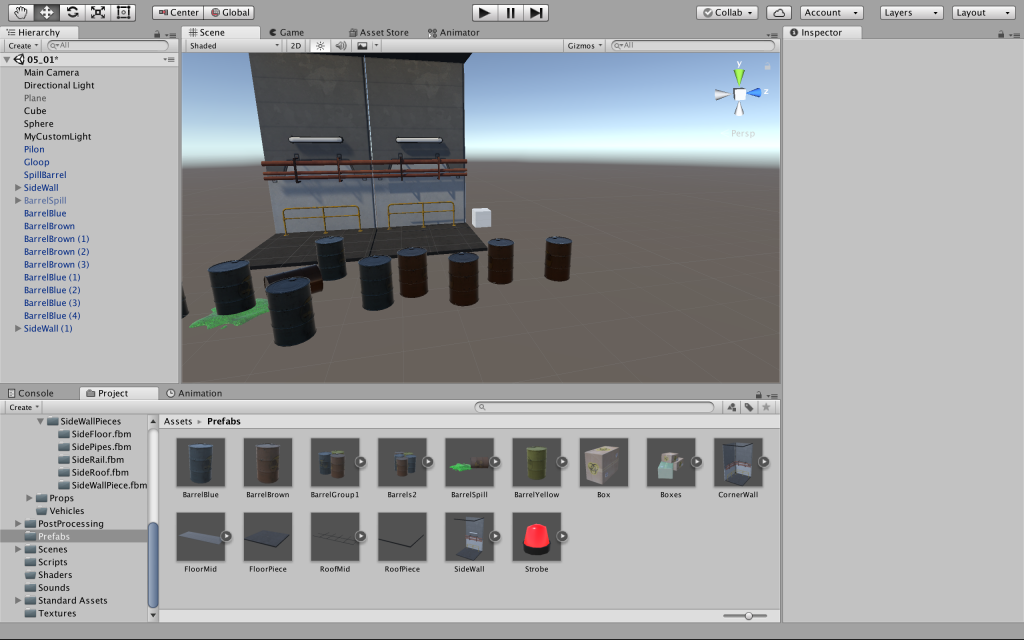Tutorial_6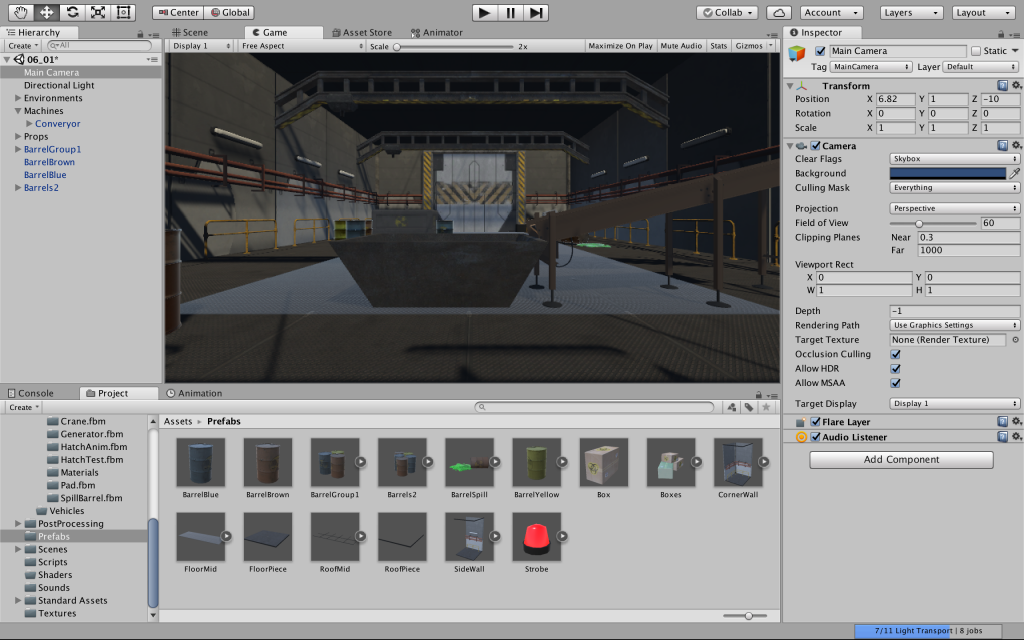Tutorial_7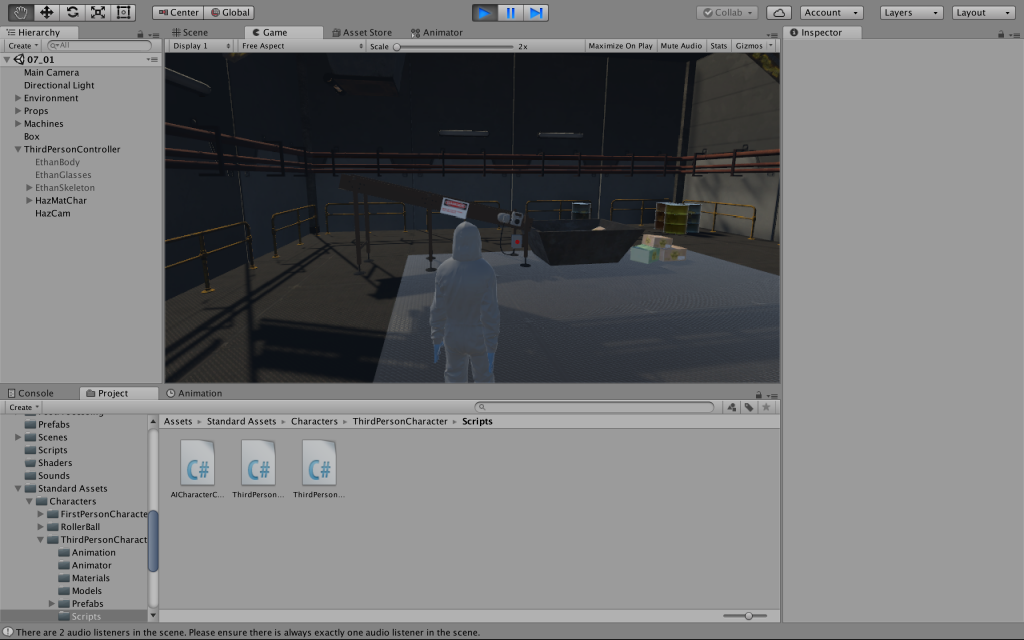Tutorial_8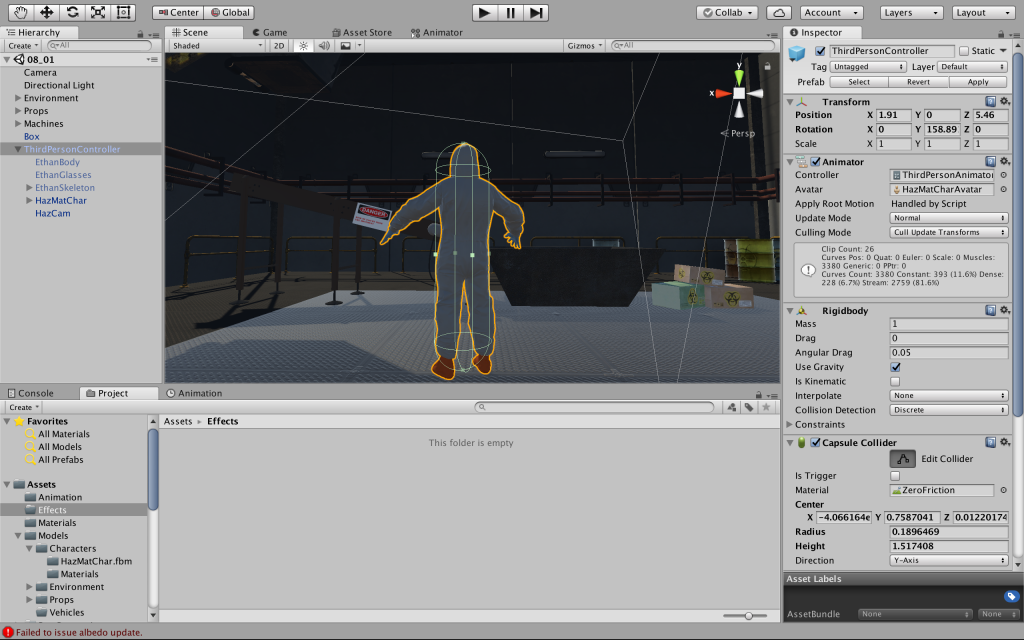Tutorial_9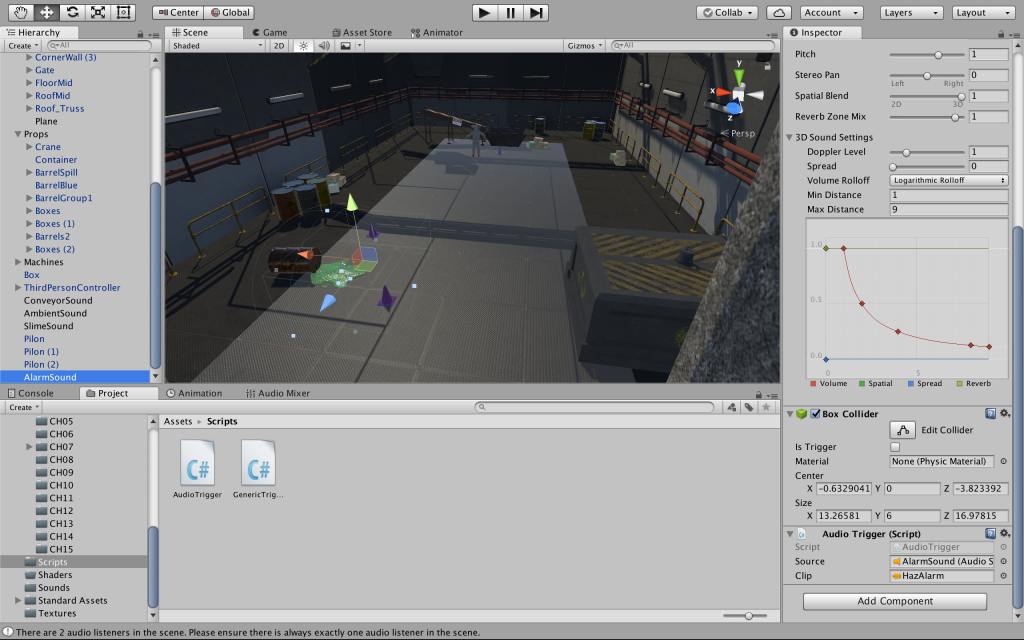Tutorial_10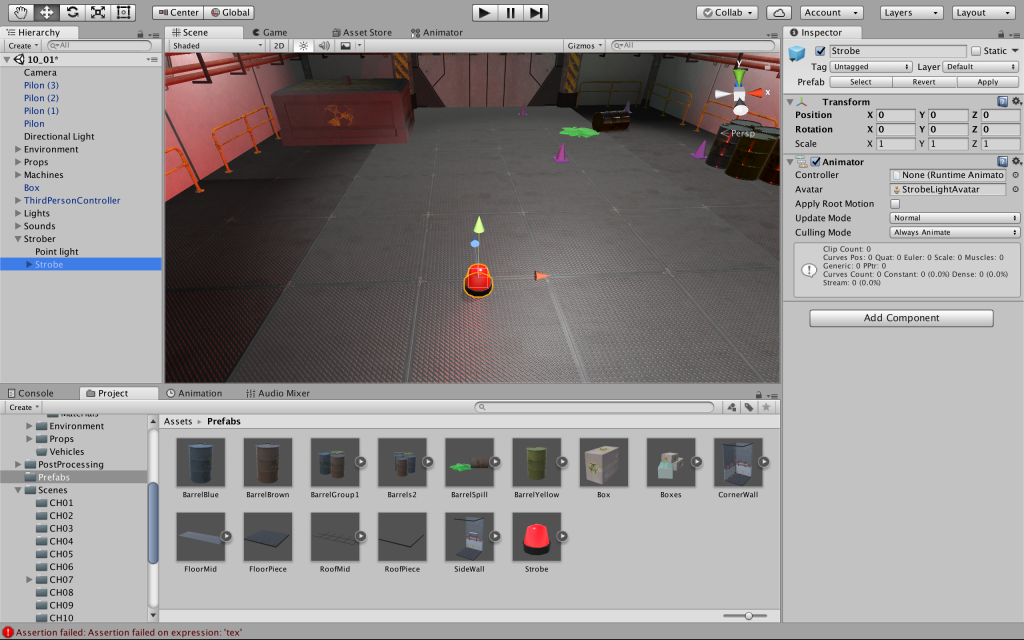Tutorial_11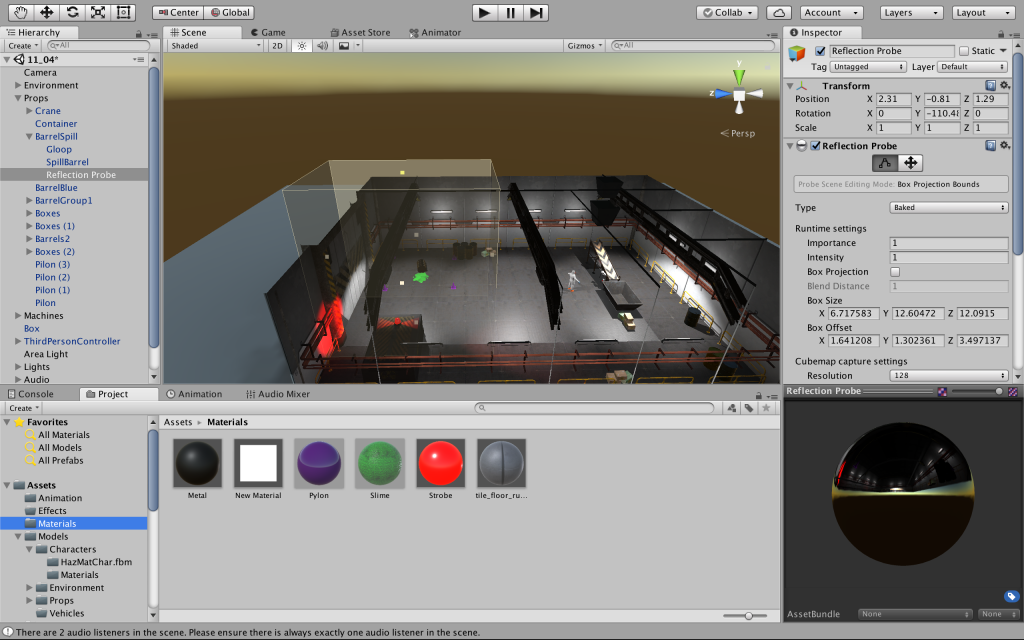Tutorial_12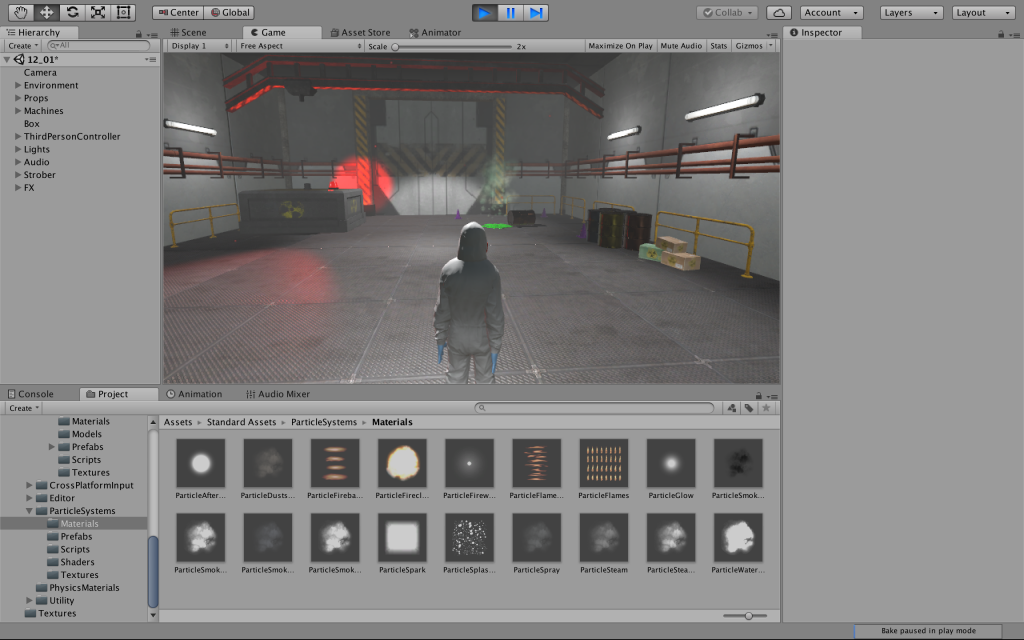Tutorial_13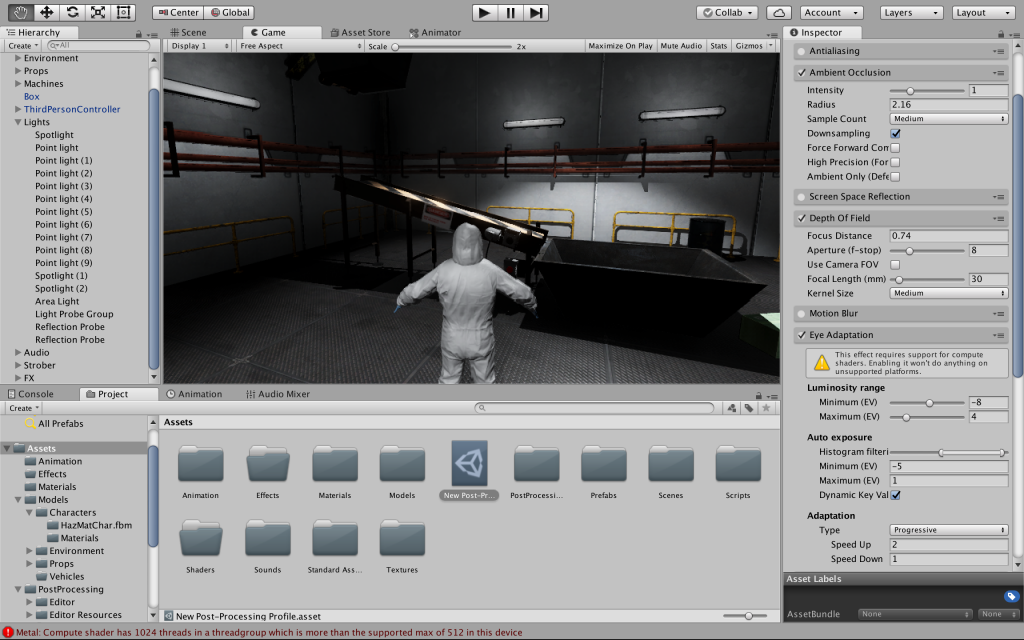Tutorial_14Tutorial_15